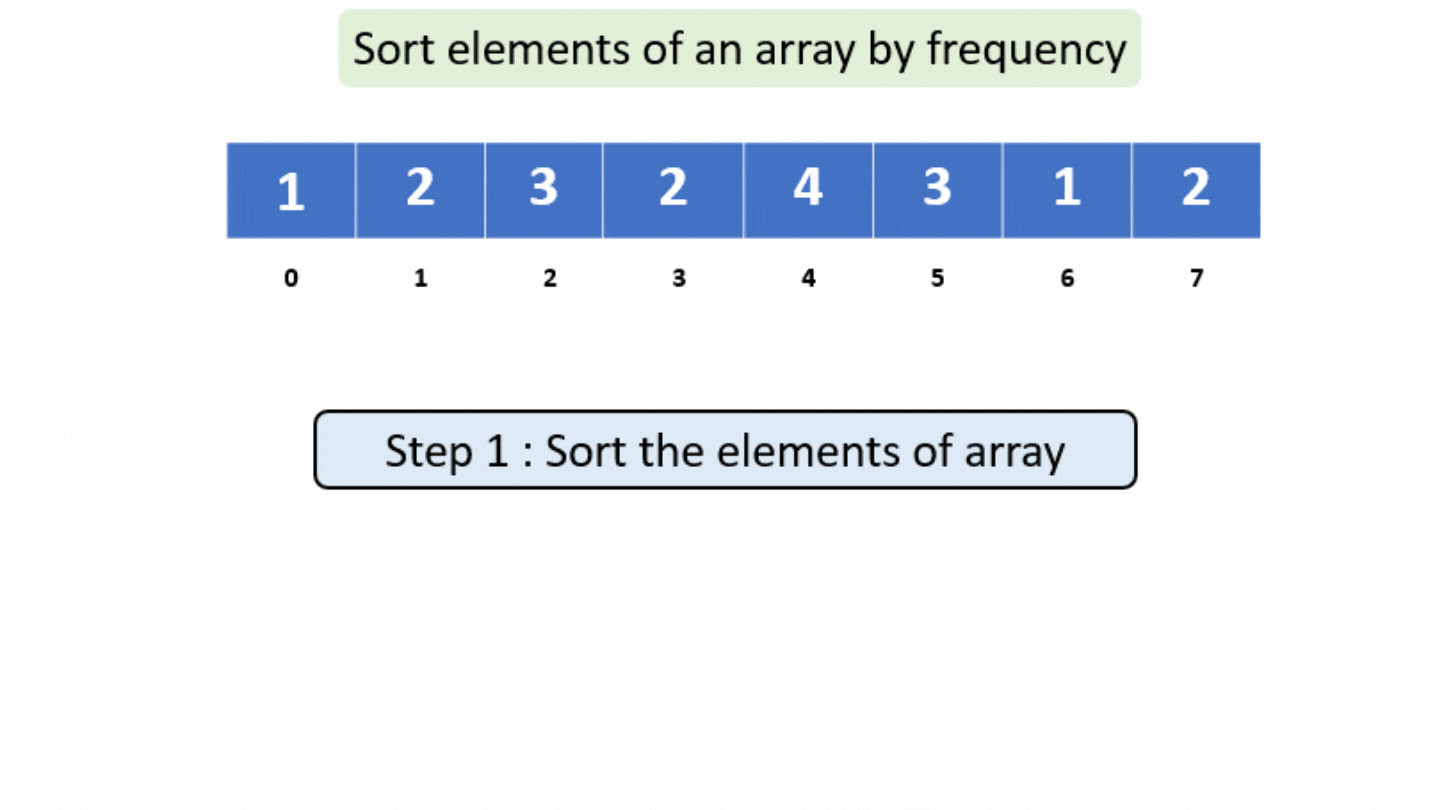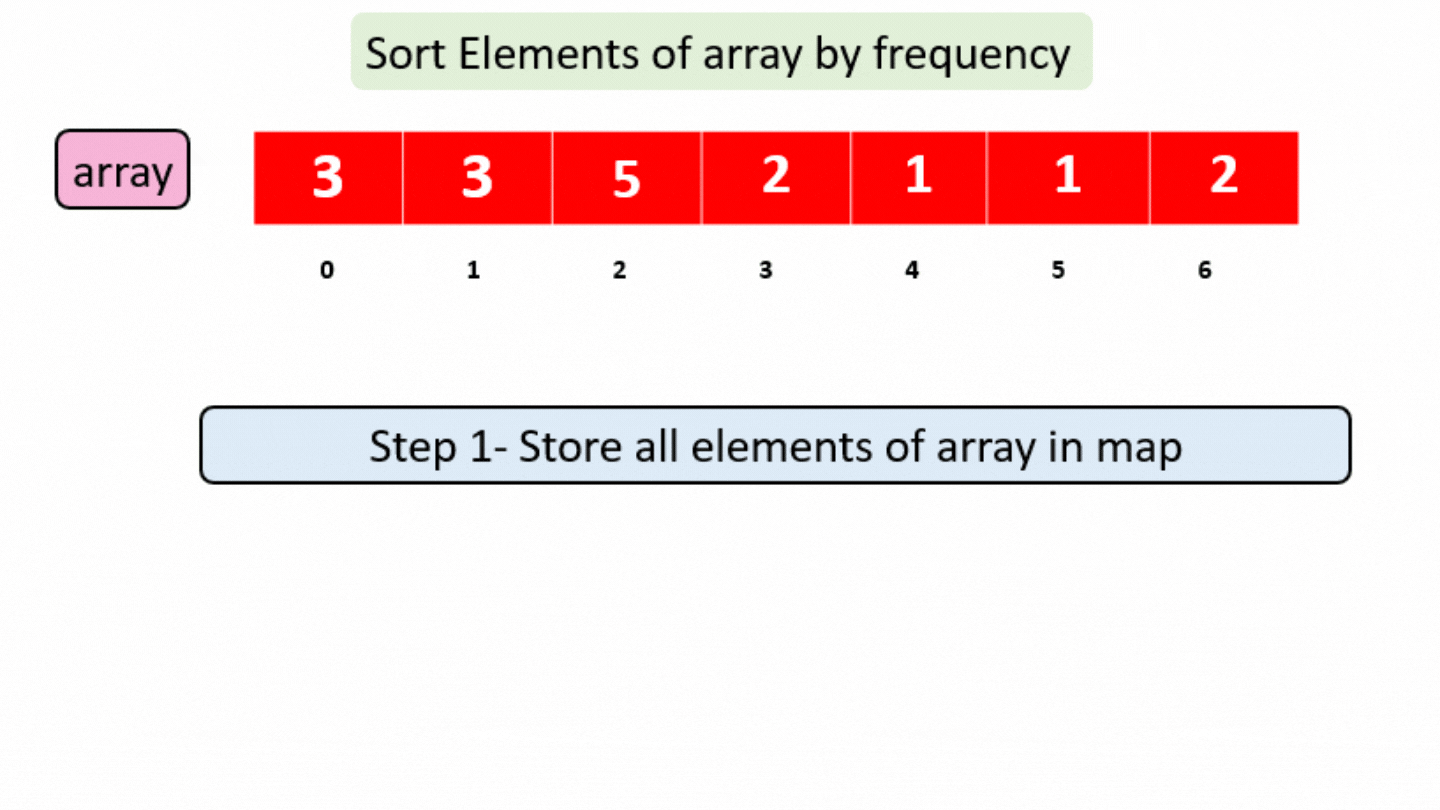# Sort Elements of an Array by Frequency

Sort Elements of an Array by Frequency

Problem Statement: Given an array of integers, having some duplicate elements, sort the array by frequency.

Examples:

```Example 1:
Input: N = 8, array[] = {1,2,3,2,4,3,1,2}
Output: 2 2 2 1 1 3 3 4
Explanation: Since  2 is present 3 times in an array , so print it 3 times ,then print ‘1’ 2 times and then ‘3’ 2 times and 4 has least frequency, it will be printed at last.

Example 2:
Input: N = 6, array[] = {-199,6,7,-199,3,5}
Output: -199 -199 3 5 6 7
Explanation: Since -199 is present 2 times so it will be printed at first , then 3 , 5 ,6 ,7 are present once in array , so print them in their sorted order.
```

### Solution

Disclaimer: Don’t jump directly to the solution, try it out yourself first.

Solution 1:

ApproachBrute Force

Step 1: Sort the given array.

Step 2: Now create a 2d array having the first row for storing distinct elements and a second row for maintaining their frequency.

Step 3: Now sort the elements according to their frequency and if two elements are of the same frequency remain them in the sorted order.Code:

## C++ Code

``````#include <iostream>
#include <algorithm>
using namespace std;
// Function to sort 2d array by frequency
void sort2darray(int array2d, int k)
{
for (int i = 0; i < k - 1; i++)
{
for (int j = 0; j < k - 1 - i; j++)
{
if (array2d[j] < array2d[j + 1])
{
swap(array2d[j], array2d[j + 1]);
swap(array2d[j], array2d[j + 1]);
}
}
}
}
// Function to sort elements of array by frequency
void Sortele(int arr[], int n)
{
// Step 1: Sort the array
sort(arr, arr + n);
// Step 2: Create a 2d array
int arr2d;
int k = 0;
int count = 0;
for (int i = 0; i < n; i++)
{
if (i == 0)
{
arr2d[k] = arr[i];
count = 1;
}
else if (arr[i] == arr[i - 1])
{
count++;
}
else
{
arr2d[k] = count;
count = 1;
k++;
arr2d[k] = arr[i];
}
}
arr2d[k] = count;
k++;

// Step 3: sort the 2d array according to frequency
sort2darray(arr2d, k);

// Step 4: Store the answer in original array
k = 0;
int i = 0;
while (i < n)
{
while (arr2d[k] > 0)
{
arr[i] = arr2d[k];
i++;
arr2d[k]--;
}
k++;
}
}
int main()
{
int n = 8;
int arr[] = {1, 2, 3, 2, 4, 3, 1, 2};
Sortele(arr, n);
for (int i = 0; i < n; i++)
{
cout << arr[i] << " ";
}
return 0;
}
``````

Output:

2 2 2 1 1 3 3 4

Time Complexity: O(n^2)

Space Complexity: O(n)

## Java Code

``````import java.util.*;
public class Main {
// Function to swap elements
public static void swap(int[][] arr, int i, int j) {
int temp1 = arr[i];
arr[i] = arr[j];
arr[j] = temp1;

int temp2 = arr[i];
arr[i] = arr[j];
arr[j] = temp2;
}
// Function to sort 2d array by frequency
public static void sort2darray(int[][] array2d, int k) {
for (int i = 0; i < k - 1; i++) {
for (int j = 0; j < k - 1 - i; j++) {
if (array2d[j] < array2d[j + 1]) {
swap(array2d, j, j + 1);
}
}
}
}
// Function to sort elements of array by frequency
public static void Sortele(int[] arr, int n) {
// Step 1: Sort the array
Arrays.sort(arr, 0, n);
// Step 2: Create a 2d array
int[][] arr2d = new int;
int k = 0;
int count = 0;
for (int i = 0; i < n; i++) {
if (i == 0) {
arr2d[k] = arr[i];
count = 1;
} else if (arr[i] == arr[i - 1]) {
count++;
} else {
arr2d[k] = count;
count = 1;
k++;
arr2d[k] = arr[i];
}
}
arr2d[k] = count;
k++;

// Step 3: sort the 2d array according to frequency
sort2darray(arr2d, k);

// Step 4: Store the answer in original array
k = 0;
int i = 0;
while (i < n) {
while (arr2d[k] > 0) {
arr[i] = arr2d[k];
i++;
arr2d[k]--;
}
k++;
}
}
public static void main(String args[]) {
int n = 8;
int[] arr = {1,2,3,2,4,3,1,2};
Sortele(arr, n);
for (int i = 0; i < n; i++) {
System.out.print(arr[i] + " ");
}
}
}
``````

Output:

2 2 2 1 1 3 3 4

Time Complexity: O(n^2)

Space Complexity: O(n)

Solution 2: Optimal Approach (using hashing and sorting)

Approach:

STEP 1: Just store all elements on a map according to their count.

STEP 2: Store all elements of the map in a vector one by one.

STEP 3: Sort that vector according to its frequency.

STEP 4: Now print them or push them into the answer vector.Code:

## C++ Code

``````#include <bits/stdc++.h>
using namespace std;
bool sortele(pair<int, int> a, pair<int, int> b)
{
if (a.second == b.second)
return a.first < b.first;
return a.second > b.second;
}
void Sortelementsbyfreq(int arr[], int n)
{
unordered_map<int, int> map;
for (int i = 0; i < n; i++)
{
map[arr[i]]++;
}
vector<pair<int, int>> vec;
for (auto it = map.begin(); it != map.end(); it++)
{
vec.push_back({it->first, it->second});
}
sort(vec.begin(), vec.end(), sortele);
for (int i = 0; i < vec.size(); i++)
{
while (vec[i].second > 0)
{
cout << vec[i].first << " ";
vec[i].second--;
}
}
cout << endl;
}
int main()
{
int arr[] = {3, 3, 5, 2, 1, 1, 2};
int n = 7;
Sortelementsbyfreq(arr, n);
return 0;
}
``````

Output: 1 1 2 2 3 3 5

Time Complexity – O(N) where N is the number of elements in the array

Space Complexity – O(N) for storing the elements in Map, vector

## Java Code

``````import java.util.*;
class Pair{
int first;
int second;
Pair(int first,int second)
{
this.first=first;
this.second=second;
}
Pair()
{

}
}
class TUF{
static void Sortelementsbyfreq(int arr[], int n)
{
HashMap<Integer, Integer> map=new HashMap<>();
for (int i = 0; i < n; i++)
{

map.put(arr[i],map.getOrDefault(arr[i],0)+1);
}
ArrayList<Pair> vec=new ArrayList<>();
for (int x:map.keySet())
{
}
Collections.sort(vec,(a,b)->{
if(a.second==b.second)
return a.first-b.first;
else
return b.second-a.second;
});
for (int i = 0; i < vec.size(); i++)
{
while (vec.get(i).second > 0)
{
System.out.print(vec.get(i).first+" ");
vec.get(i).second--;
}
}

}
public static void main(String args[])
{
int arr[] = {3, 3, 5, 2, 1, 1, 2};
int n = 7;
Sortelementsbyfreq(arr, n);

}
}``````

Output: 1 1 2 2 3 3 5

Time Complexity – O(N) where N is the number of elements in the array

Space Complexity – O(N) for storing the elements in Map, vector

Special thanks to Gurmeet Singh for contributing to this article on takeUforward. If you also wish to share your knowledge with the takeUforward fam, please check out this article